# AP Physics 1 Multiple-Choice Practice Test 25

### Test Information10 questions18 minutes

1. A sphere starts from rest atop a hill with a constant angle of inclination and is allowed to roll without slipping down the hill. What force provides the torque that causes the sphere to rotate?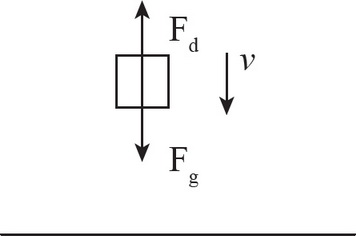2. Which of the following correctly describes the motion of a real object in free fall? Assume that the object experiences drag force proportional to speed and that it strikes the ground before reaching terminal speed.

3. Which of the following concerning uniform circular motion is true?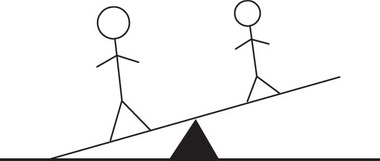4. A girl of mass m and a boy of mass 2m are sitting on opposite sides of a lightweight seesaw with its fulcrum in the center. Right now, the boy and girl are equally far from the fulcrum, and it tilts in favor of the boy. Which of the following would NOT be a possible method to balance the seesaw?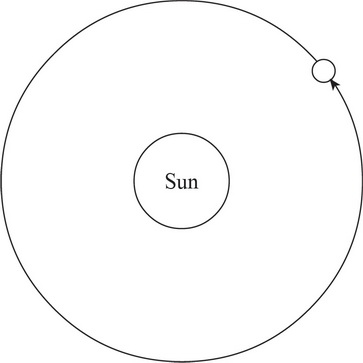5. Earth's orbit around the Sun can be approximated as a circle. Given that the Earth's mass is m, its tangential speed as it revolves around the Sun is v, and the distance from the Sun to the Earth is r, which of the following correctly describes the work done by the centripetal force, Wc, in one year's time?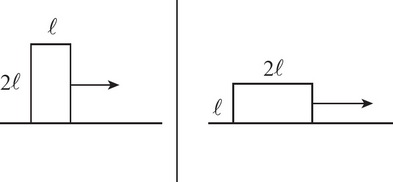6. A wooden block experiences a frictional force, Ff, as it slides across a table. If a block of the same material with half the height and twice the length were to slide across the table in the same direction, what would be the frictional force it experienced?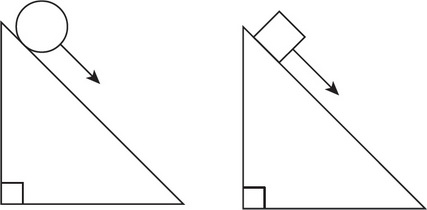7. Two objects, a sphere and a block of the same mass, are released from rest at the top of an inclined plane. The sphere rolls down the inclined plane without slipping. The block slides down the plane without friction. Which object reaches the bottom of the ramp first?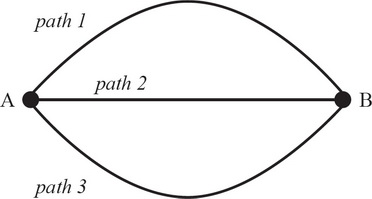8. In the diagram above, each of the three frictionless tracks shown here contains an object of mass m. Each object starts at point A with the same initial horizontal velocity v0, which in each case is sufficient to allow the object to reach the end of the track at point B. (Path 1 is directed up, path 2 is directed horizontally, and path 3 is directed down.) The masses remain in contact with the tracks throughout their motions. The displacement A to B is the same in each case, and the total path lengths of path 1 and 3 are equal. If t1, t2, and t3 are the total travel times between A and B for paths 1, 2, and 3, respectively, what is the relation among these times?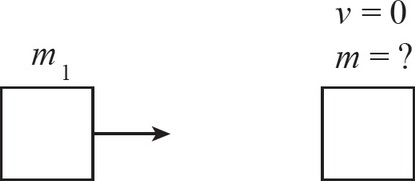9. An object of mass m1 experiences a linear, elastic collision with a stationary object of unknown mass. In addition to m1, what is the minimum necessary information that would allow you to determine the mass of the second object?

10. A block is dragged along a table and experiences a frictional force, f, that opposes its movement. The force exerted on the block by the table is